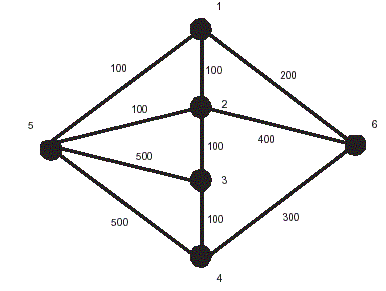Online JudgeProblem SetAuthorsOnline ContestsUser
Web Board
Home Page
F.A.Qs
Statistical Charts
Problems
Submit Problem
Online Status
Prob.ID:
Register
Update your info
Authors ranklist
Current Contest
Past Contests
Scheduled Contests
Award Contest
 User ID: Password:
Register
Language:
The Longest Detour Problem
 Time Limit: 1000MS Memory Limit: 10000K Total Submissions: 692 Accepted: 230

Description

Let G be a weighted graph, i.e., every edge in G is associated with a nonnegative integer weight. The length of a path is the sum of edge weights in the path. A shortest path between vertices r and s in G, denoted by PG(r, s), is defined as a path with the shortest length from r to s. The distance between vertices r and s, denoted by dG(r, s), is the length of the shortest path PG(r, s). For two vertices in a connected graph, there exists at least one shortest path between them. Let e = (u, v) be an edge in PG(r, s) with v closer to s than u (v may be s). Let G - e denote the subgraph obtained by removing edge e from G. A detour from u is the shortest path from u to s in G - e, or PG-e(u, s). Edge e is a detour -critical edge in PG(r, s) if the removal of e results in the maximum distance increment from u to s. In other words, if e is a detour -critical edge in PG(r, s), then dG-e(u, s) - dG(u, s) is maximum among all edges in PG(r, s). The longest detour problem is to find the maximum distance increment of a shortest path.Figure 4: A weighted graph G.

For example, see Figure 4. PG(4, 1) =< 4, 3, 2, 1 > is the shortest path from vertex 4 to vertex 1. Path < 4, 6, 1 > is the detour from vertex 4 to vertex 1 if edge (4, 3) is removed. Path < 3, 5, 1 > is the detour from vertex 3 to vertex 1 if edge (3, 2) is removed. Path < 2, 5, 1 > is the detour from vertex 2 to vertex 1 if edge (2, 1) is removed. The detour -critical edge in PG(4, 1) is not edge (4, 3) or edge (2, 1) but edge (3, 2) since dG-(3,2)(3, 1) - dG(3, 1) = 600 - 200 = 400 is greater than dG-(4,3)(4, 1) - dG(4, 1) = 500 - 300 = 200 and dG-(2,1)(2, 1) - dG(2, 1) = 200 - 100 = 100.

The algorithm for finding detours, as well as determining the detour-critical edges, is important from the viewpoint of network management. Due to a sudden edge failure

from some vertex, the message must be retransmitted through a detour from the vertex adjacent to the faulty edge.

Suppose that we have several networks. Each network is connected and contains at most n vertices, where 3 <= n <= 100. Assume now that you are hired to serve as a

network administrator and have to determine the maximum distance increment caused by a detour-critical edge of a given shortest path for each network.

Input

The input consists of several test cases. The first line contains an integer indicating the number of test cases. Each test case starts with a positive integer n, where 3 <= n <= 100. The following n lines represent the adjacency matrix of a network. An adjacency matrix of a network G with n vertices, denoted by A(G) = [wu,v], is an n * n matrix such that wu,v > 0 if (u, v) is an edge of G, and wu,v= 0 otherwise, where wu,v in a nonnegative integer. Note that any two elements in each line of an adjacency matrix are separated by a space. The last line of each test case represents the sequence of vertices in a given shortest path in which there is also a space between two vertices. Note that the first and the last vertices denote the source and the destination vertices, respectively. For example, the adjacency matrix of the graph in Figure 4 is shown in test case 3 of the sample input.

Output

For each test case, output the maximum distance increment caused by the detour-critical edge of the given shortest path in one line.

Sample Input

```3
3
0 10 20
10 0 10
20 10 0
3 2 1
4
0 10 10 30
10 0 30 0
10 30 0 10
30 0 10 0
4 3 1 2
6
0 100 0 0 100 200
100 0 100 0 100 400
0 100 0 100 500 0
0 0 100 0 500 300
100 100 500 500 0 0
200 400 0 300 0 0
4 3 2 1```

Sample Output

```20
30
400```

Source

[Submit]   [Go Back]   [Status]   [Discuss]Home PageGo BackTo top

All Rights Reserved 2003-2013 Ying Fuchen,Xu Pengcheng,Xie Di
Any problem, Please Contact Administrator